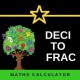# Typescript EPSILON & Floating point precision

In this tutorial, we will explain the Typescript `Number.EPSILON` property. It represents the difference between 1 and the smallest floating-point number greater than 1. It exists because not all decimal numbers can be represented accurately and exactly in the binary system.

## Floating-point precision problem

The computers use the binary system to store numbers and use the floating-point arithmetic, while in real-world we use the decimal system. Not all decimal numbers can be represented correctly in binary numbers.

For Example

`0.1` when converted from decimal to binary results in `0.0001100110011001101`. Now convert it back from binary to decimal will result in `0.1000003814697265625`

The above case is similar to how 1/3 ( 0.33333) cannot be represented as accurately in decimal.

The decimal 10 is represented as 1010 in binary. Now divide 1 by 1010 and you will find out that the division goes on forever just like 1/3.

Because of the above loss of precision occurs, the strange results like the following happens

The difference is very minute, but this definitely causes a problem when you compare two results. For Example, the following results in `false` rather than `true`.

## Number.EPSILON

One of the ways in which to avoid such problems is to use a very small number as a tolerance for comparison. If the difference between the compared numbers is less than the tolerance, then they are considered equal.

The typescript provides `Number.EPSILON` static property of the `Number` object as predefined tolerance. It is the difference between 1 and the smallest floating-point number greater than 1.

If the difference between the two numbers is less than the `Number.EPSILON`, then they are considered equal.

## Summary

The conversion from a decimal number to binary is not always accurate. It can result in loss of precision. It is evident when you compare two number (ex: `(0.1 + 0.2) ==.3`), who are supposed to be equal but results in false. `Number.EPSILON` is used as tolerance for the number comparison. If the difference between the numbers is less than the `Number.EPSILON`, then they are considered equal.

### 1 thought on “Typescript EPSILON & Floating point precision”

1.